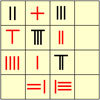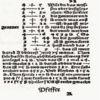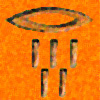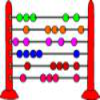# Search by Topic

Filter by: Content type:
Age range:
Challenge level:

### There are 11 results

Broad Topics > History and Philosophy of Mathematics > History of number systems### The Greedy Algorithm

##### Age 11 to 14 Challenge Level:

The Egyptians expressed all fractions as the sum of different unit fractions. The Greedy Algorithm might provide us with an efficient way of doing this.### Keep it Simple

##### Age 11 to 14 Challenge Level:

Can all unit fractions be written as the sum of two unit fractions?### Egyptian Fractions

##### Age 11 to 14 Challenge Level:

The Egyptians expressed all fractions as the sum of different unit fractions. Here is a chance to explore how they could have written different fractions.### The Development of Algebra - 2

##### Age 11 to 18

This is the second article in a two part series on the history of Algebra from about 2000 BCE to about 1000 CE.### The History of Negative Numbers

##### Age 11 to 18

This article -useful for teachers and learners - gives a short account of the history of negative numbers.### Negative Numbers

##### Age 11 to 14

A brief history of negative numbers throughout the ages### Mathematical Symbols

##### Age 5 to 14

A brief article written for pupils about mathematical symbols.### History of Fractions

##### Age 7 to 14

Who first used fractions? Were they always written in the same way? How did fractions reach us here? These are the sorts of questions which this article will answer for you.### Pi, a Very Special Number

##### Age 7 to 14

Read all about the number pi and the mathematicians who have tried to find out its value as accurately as possible.### Stone Age Counting

##### Age 5 to 7 Challenge Level:

What could these drawings, found in a cave in Spain, represent?### Fibonacci's Three Wishes 1

##### Age 7 to 14

First or two articles about Fibonacci, written for students.